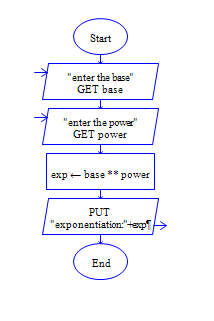# Python Exponentiation

Python program to calculate Power of a Number

Sample Input 1:

2 3

Sample Output 1 :

8

#### Flow Chart DesignStrongly recommended to Solve it on your own, Don't directly go to the solution given below.

#### Program or Solution

``` b=int(input("enter the base:")) p=int(input("Enter the power:")) exp=b**p print("exponentiation : {}".format(exp)) ```

#### Program Explanation

Read two inputs using input() method.

Convert it into integer by int() method.

% operator gives remainder.